### Home > CALC > Chapter 6 > Lesson 6.1.3 > Problem6-35

6-35.
1. Examine the integrals below. Consider the multiple tools available for integrating and use the best strategy. After evaluating each integral, write a short description of your method. Homework Help ✎

1.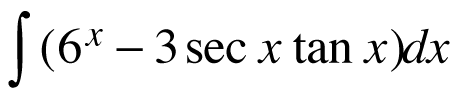2.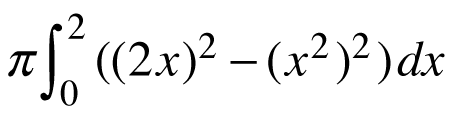3.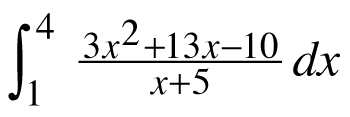4.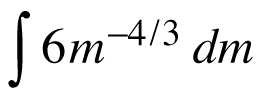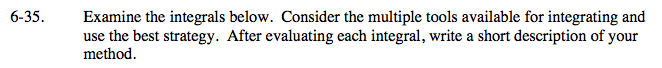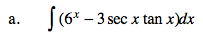What two functions have derivative of 6x and 3sec(x)tan(x), respectively?

$\frac{6^{x}}{\text{ln} 6}-3\text{sec}x+C$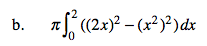(2x)2 − (x2)2 = 4x2x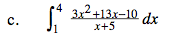Factor.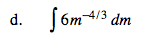Undo the Power Rule.